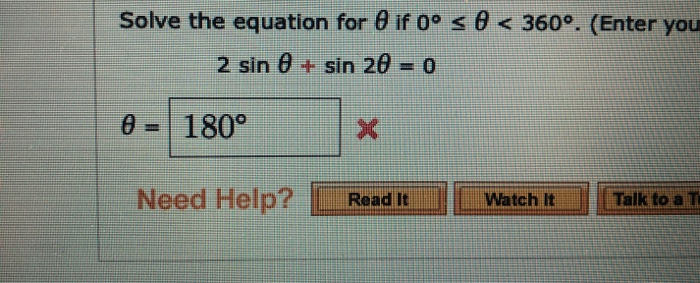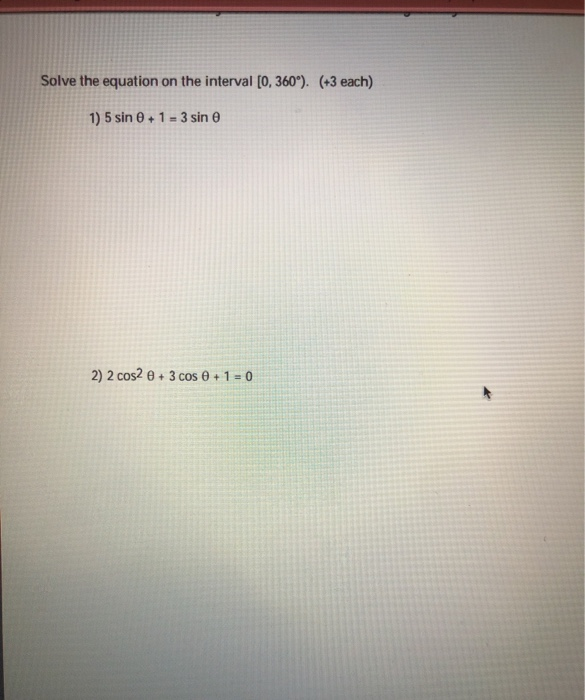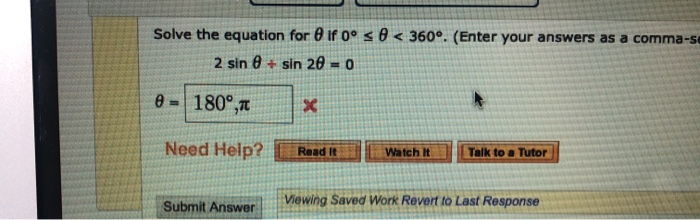# solve the equation sin(3x) + (1/2) = 2cos^2(x) for X to be between 0 and 360

solve the equation
sin(3x) + (1/2) = 2cos^2(x)
for X to be between 0 and 360

Use the relation
cos²(x)=1-sin²(x)
to convert the equation to a quadratic in sin(x).

Solve for the value of sin(x), say s1 and s2 and reject any impossible roots such as |s|>1.

For the remaining root(s), solve for
sin(θ)=s. There should always be two values of θ (0≤ θ<360°)for each valid value of s, where -1≤s≤+1.

If you need further help, post.
##### Add Answer of: solve the equation sin(3x) + (1/2) = 2cos^2(x) for X to be between 0 and 360
Similar Homework Help Questions
• ### Solve the following equation for 0 less than and/or equal to "x" less than and/or equal to 360 -- cos^2x - 1 = sin^2x -- Attempt: cos^2x - 1 - sin^2x = 0 cos^2x - 1 - (1 - cos^2x) = 0 cos^2x - 1 - 1 + cos^2x = 0 2cos^2x - 2 = 0 (2cos^2x/2)= (-2/2) cos^

Solve the following equation for 0 less than and/or equal to "x" less than and/or equal to 360--cos^2x - 1 = sin^2x--Attempt:cos^2x - 1 - sin^2x = 0cos^2x - 1 - (1 - cos^2x) = 0cos^2x - 1 - 1 + cos^2x = 02cos^2x - 2 = 0(2cos^2x/2)= (-2/2)cos^2x = -1cosx = square root of -1And I can't do anything with this now...what am I doing wrong?--Textbook answers:0, 180, 360--

• ### Solve the equation for @ if 0° s @ < 360°. (Enter you 2 sin 0...Solve the equation for @ if 0° s @ < 360°. (Enter you 2 sin 0 + sin 20 = 0 0 = 180° Need Help? Read it Watch It Talk to al

• ### Solve each equation for 0 is less than and equal to "x" is less than and equal to 360 3sinx = 2cos^{2}x ----- I don't know how to solve this equation

Solve each equation for 0 is less than and equal to "x" is less than and equal to 3603sinx = 2cos^{2}x-----I don't know how to solve this equation...this is what I have, but I don't know if I'm on the right track or not3sinx = 1 - 2sin^{2}x3sinx - 1 + 2sin^{2}x = 02sin^{2}x + 3sinx - 1 = 0sinx(3 + 2sinx) = 1...and if I am, what do I do next now?-----The answers are:30 and 150 degrees

• ### Solve the equation: sin (3x+30°) =√3/2 for 0°≤x≤90°

Solve the equation: sin (3x+30°) =√3/2 for 0°≤x≤90°

• ### Solve the equation on the interval [0, 360°). (43 each) 1) 5 sin 0+1 = 3...Solve the equation on the interval [0, 360°). (43 each) 1) 5 sin 0+1 = 3 sin 2) 2 cos2 + 3 cos 0 + 1 = 0

• ### Solve the equation in the interval [0°, 360°). sin^2θ - sin θ - 12 = 0...

Solve the equation in the interval [0°, 360°). sin^2θ - sin θ - 12 = 0 sin 2θ = -sin θ 2 cos2θ + 7 sin θ = 5

• ### Solve the equation in the interval [0°, 360°). 4 sin^2θ = 3 csc θ = 1...

Solve the equation in the interval [0°, 360°). 4 sin^2θ = 3 csc θ = 1 + cot θ 3 sin^2θ - sin θ - 4 = 0 2 cos^3θ = cos θ

• ### how do i solve sin(x)+2cos(2x)=0

how do i solve sin(x)+2cos(2x)=0

• ### Solve: 2 sin x - 1 = 0 for 0 degrees is less than or equal to x is less than 360 degrees

Solve: 2 sin x - 1 = 0 for 0 degrees is less than or equal to x is less than 360 degrees.

• ### Solve the equation for @ if 0° s @ < 360°. (Enter your answers as a...Solve the equation for @ if 0° s @ < 360°. (Enter your answers as a comma-se 2 sin 0 + sin 20 = 0 0 = 180°, X Need Help? Read It Watch It Talk to a Tutor Submit Answer Viewing Saved Work Revert to Last Response

Free Homework App